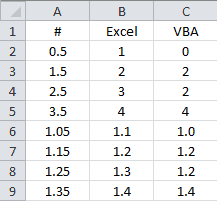## Introduction

Hey, guys! I just made it back from an incredible Alaskan vacation, and I’m all refreshed and ready to give you more exciting VBA tutorials.Here goes!

The VBA Round function behaves differently than the Excel Worksheet ROUND function. This tutorial tells you everything you need to know about the differences between the two rounding functions.

It’s important to understand the differences BEFORE you begin a project so you won’t spend hours performing unnecessary troubleshooting.

## VBA Round vs Excel ROUND

### 1st Difference: Required Arguments

The VBA Round function and the Excel ROUND function follow the same syntax, with a few subtle differences.

Excel: =ROUND(number, num_digits)
VBA: Round(Number, [NumDigitsAfterDecimal])

The Excel ROUND function requires two arguments. The first argument is the number you want to round and the second argument is a whole number greater than or equal to 0 representing how many digits after the decimal you want to round to. Both arguments are required.

The VBA Round function accepts the same two arguments, but the second argument is optional. If you omit the NumDigitsAfterDecimal argument, the Round function in VBA assumes you want to round to the nearest whole number. In other words, it defaults to 0 decimal digits.

### 2nd Difference: Rounding Methods

You’re probably familiar with the Excel ROUND function. It rounds up for decimals ending in a 5 or greater. For example,

```Excel Rounding
ROUND(1.5,0)  equals 2
ROUND(1.4,0)  equals 1
ROUND(2.5,0)  equals 3
ROUND(2.4,0)  equals 2
ROUND(2.25,1) equals 2.3
ROUND(2.24,1) equals 2.2
```

This is how most people were typically taught to round.

The VBA Round function is different. In VBA, the round function uses what is often referred to as Banker’s Rounding. Bankers rounding rounds decimals ending in a 5 to the nearest even number. Let’s take a look at some examples:

```VBA Rounding
Round(1.5)    equals 2
Round(1.4)    equals 1
Round(2.5)    equals 2
Round(2.4)    equals 2
Round(2.25,1) equals 2.2
Round(2.24,1) equals 2.2
```

Make powerful macros with our free VBA Developer Kit

It’s easy to copy and paste a macro like this, but it’s harder make one on your own. To help you make macros like this, we built a free VBA Developer Kit and wrote the Big Book of Excel VBA Macros full of hundreds of pre-built macros to help you master file I/O, arrays, strings and more - grab your free copy below.

I'll take a free VBA Developer Kit

Notice how each time the digit you wanted to round ended in a 5, the VBA round function rounded to the nearest even integer, regardless of whether that required rounding up or rounding down. Also notice how the second argument is optional when you want to round to the nearest whole number.

I want to show you a side by side example comparing the results of Excel rounding and VBA rounding to drive home the differences.Here’s the macro I used to populate the VBA results in the image above:

``````Sub VBAroundDemo()
For i = 2 To 5
Range("c" & i) = Round(Range("a" & i), 0)
Next i
For i = 6 To 9
Range("c" & i) = Round(Range("a" & i), 1)
Next i
End Sub``````

## How to use Excel ROUND in VBA

The advantage of banker’s rounding is that it attempts to remove any bias in calculations performed on rounded numbers. Many people are unfamiliar with this way of rounding since it’s different from what they were taught and they assume that there must be an error in the VBA rounding algorithm. This isn’t true, but the perception can cause hours of frustration to user’s not expecting the behavior difference.

Don’t despair. You can still use the more traditional rounding algorithm in VBA by calling the Excel ROUND function with Application.WorksheetFunction. Here’s how you do it:

``````Sub ExcelROUNDdemo()
Dim d As Double
d = 2.5
d = Application.WorksheetFunction.Round(d, 0) 'Now returns a value of 3
End Sub``````

In the example above, the variable d is rounded to a value of 3 when you use the Excel Worksheet ROUND function. If you were to use the VBA round function, `d = Round(d, 0)`, it would return a value of 2.

## Closing Thoughts

I hope you learned something in this tutorial. Although there are more than one difference between the VBA Round function and the Excel ROUND function, the key takeaway is that the two functions use different rounding algorithms. Be aware of this to avoid surprises when you’re deep into your next project.

That’s all for this tutorial. When you’re ready to take your VBA to the next level, subscribe using the form below.